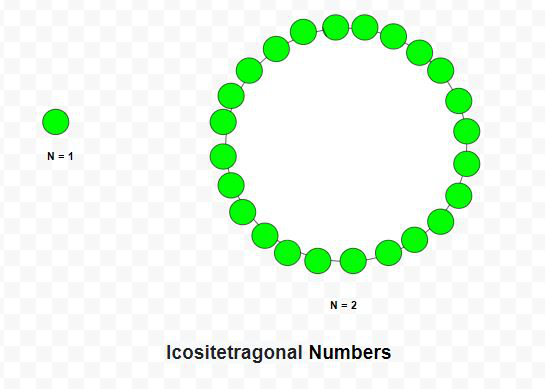# Icositetragonal Number

Given a number N, the task is to find the Nth Icositetragonal number.

An Icositetragonal number is a class of figurate number. It has a 24-sided polygon called Icositetragon. The N-th Icositetragonal number count’s the number of dots and all others dots are surrounding with a common sharing corner and make a pattern.

Examples:

Input: N = 2
Output: 24

Input: N = 6
Output: 336

## Recommended: Please try your approach on {IDE} first, before moving on to the solution.Approach: The Nth icositetragonal number is given by the formula:

``````

Below is the implementation of the above approach:

## C++

 `// C++ program to find nth ` `// Icositetragonal number ` ` `  `#include ` `using` `namespace` `std; ` ` `  `// Function to find ` `// Icositetragonal number ` `int` `Icositetragonal_num(``int` `n) ` `{ ` `    ``// Formula to calculate nth ` `    ``// Icositetragonal number ` `    ``return` `(22 * n * n - 20 * n) / 2; ` `} ` ` `  `// Driver Code ` `int` `main() ` `{ ` `    ``int` `n = 3; ` ` `  `    ``cout << Icositetragonal_num(n) << endl; ` ` `  `    ``n = 10; ` ` `  `    ``cout << Icositetragonal_num(n); ` ` `  `    ``return` `0; ` `} `

## Java

 `// Java program to find nth ` `// icositetragonal number ` `import` `java.util.*; ` ` `  `class` `GFG { ` `     `  `// Function to find ` `// icositetragonal number ` `static` `int` `Icositetragonal_num(``int` `n) ` `{ ` `     `  `    ``// Formula to calculate nth ` `    ``// icositetragonal number ` `    ``return` `(``22` `* n * n - ``20` `* n) / ``2``; ` `} ` ` `  `// Driver code ` `public` `static` `void` `main(String[] args) ` `{ ` `    ``int` `n = ``3``; ` `    ``System.out.println(Icositetragonal_num(n)); ` `     `  `    ``n = ``10``; ` `    ``System.out.println(Icositetragonal_num(n)); ` `} ` `} ` ` `  `// This code is contributed by offbeat `

## Python3

 `# Python3 program to find nth  ` `# Icositetragonal number  ` ` `  `# Function to find ` `# Icositetragonal number ` `def` `Icositetragonal_num(n): ` `     `  `    ``# Formula to calculate nth  ` `    ``# Icositetragonal number  ` `    ``return` `(``22` `*` `n ``*` `n ``-` `20` `*` `n) ``/` `2` ` `  `# Driver Code ` `n ``=` `3` `print``(``int``(Icositetragonal_num(n)))  ` ` `  `n ``=` `10` `print``(``int``(Icositetragonal_num(n)))  ` ` `  `# This code is contributed by divyeshrabadiya07 `

## C#

 `// C# program to find nth ` `// icositetragonal number ` `using` `System; ` ` `  `class` `GFG{ ` `     `  `// Function to find ` `// icositetragonal number ` `static` `int` `Icositetragonal_num(``int` `n) ` `{ ` `     `  `    ``// Formula to calculate nth ` `    ``// icositetragonal number ` `    ``return` `(22 * n * n - 20 * n) / 2; ` `} ` ` `  `// Driver code ` `public` `static` `void` `Main(``string``[] args) ` `{ ` `    ``int` `n = 3; ` `    ``Console.Write(Icositetragonal_num(n) + ``"\n"``); ` `     `  `    ``n = 10; ` `    ``Console.Write(Icositetragonal_num(n) + ``"\n"``); ` `} ` `} ` ` `  `// This code is contributed by rutvik_56 `

Output:

```69
1000
```

Attention reader! Don’t stop learning now. Get hold of all the important DSA concepts with the DSA Self Paced Course at a student-friendly price and become industry ready.

My Personal Notes arrow_drop_upSmall things always make you to think big

If you like GeeksforGeeks and would like to contribute, you can also write an article using contribute.geeksforgeeks.org or mail your article to contribute@geeksforgeeks.org. See your article appearing on the GeeksforGeeks main page and help other Geeks.

Please Improve this article if you find anything incorrect by clicking on the "Improve Article" button below.

Article Tags :
Practice Tags :

Be the First to upvote.

Please write to us at contribute@geeksforgeeks.org to report any issue with the above content.